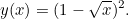# Finding the intersection envelope

We want to find the formula for the intersection between any two adjacent lines in our bridge.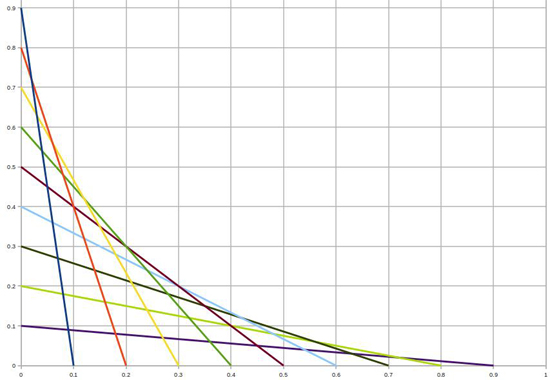Let’s look at a line that intersects the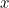axis at a certain point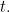Its function,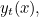is 0 at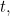and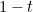at 0.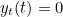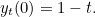The general equation for such a line is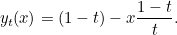Now let’s look at a line which intersects theaxis at some pointand the line just after it, which intersects theaxis at a point we call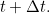The curve we are after is the one we’d get if there were infinitely many chords. In other words, as the spacing of our chords on theaxis,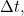approaches 0, the point in which our two lines intersect approaches a point on our envelope.

Let’s first find the intersection point of our two lines by setting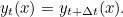This gives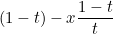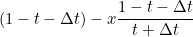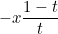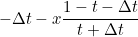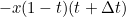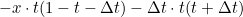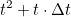As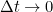we get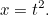Putting this in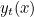we get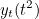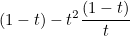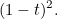So we now know that our curve is defined as all the points that satisfy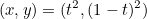for allin [0,1]. So the curve we are after is